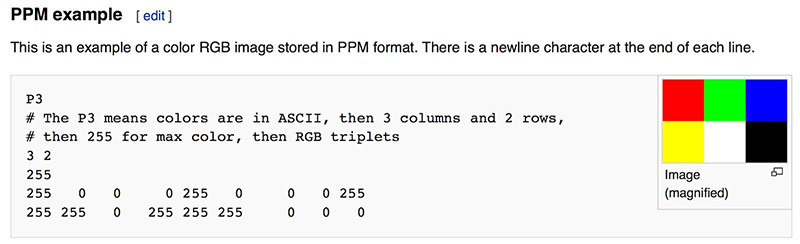0%

PlaceHolder

Github Repo

# 漫反射材质

## 一种简易的漫反射材质## 限制子射线的数量

ray_color 函数是递归的，递归出口是其与任何物体没有交点的时候，但是这可能会画上很长时间，因此需要一个最大递归深度，到达最大深度后不返回光线。## 使用 Gamma 校正获得准确的色彩强度 我们会发现图片太暗了，以至于看不清图片的阴影，需要做一些 Gamma 矫正。在此情况下，使用 Gamma 2，意味着将色彩提高 1/Gamma 次幂，即 1/2 次幂，即平方根。 ![经过 Gamma 矫正所得](/img/eleventh.png)
## 修复阴影失真 某些反射光线在不完全在 t = 0 时击中了它们正在反射的对象,而是在t=-0.0000001或t=0.00000001或任何浮点近似值与球面交点相交，所以我们需要忽视十分接近于0的浮点数。

## 真实的 Lambertian 反射random_unit_vector() 是现有的 random_in_unit_sphere() 函数的替代品。## 另一种漫反射形式Github Repo

# 抗锯齿

## 生成随机数

[dependencies]
rand = "0.7"

## 使用多个样本生成像素rtweekend.h中添加clamp(x,min,max)使得输出值在[min,max]范围内Github RepoGithub Repo$$\frac {-b \pm \sqrt {b^2-4ac}}{2a}$$

$$=\frac {-2h \pm \sqrt {(2h)^2-4ac}}{2a}$$

$$=\frac {-2h \pm 2 \sqrt {h^2-ac}}{2a}$$

$$=\frac {-h \pm \sqrt {h^2-ac}}{a}$$

Github Repo

$$(\boldsymbol{P-C}) \cdot (\boldsymbol{P-C})=R^2$$

$$(\boldsymbol P(t)-\boldsymbol C)(\boldsymbol P(t)-\boldsymbol C) = R^2$$

$$(\boldsymbol A + t\boldsymbol b)(\boldsymbol A + t\boldsymbol b) = R^2$$

$$t^2\boldsymbol b \cdot \boldsymbol b +2t \boldsymbol b \cdot (\boldsymbol A - \boldsymbol C) +(\boldsymbol A - \boldsymbol C)(\boldsymbol A - \boldsymbol C) -R^2 =0$$Github Repo

ray tracer 分为三个步骤，计算从眼睛到像素的射线，确定与射线有交点的物品，计算交点处的颜色。ray_color(r) 方法根据 r 的射线方向的 y 坐标确定白色与蓝色的混合程度，y 的值越大，越趋近于蓝色。如果将 main.rs 第十三行改成(1.0 - t) * Vec3(0.2, 0.9, 0.2) + t * Vec3(0.2, 0.0, 1.0)结果如下Github Repo

前一阵子因为兴趣看了看 Rust 的教程，感觉上手不容易，即使做完了 rustlings 里所有的练习仍感觉云里雾里。偶然在某网站上看到有人推荐这个网站，学习其内容的同时对其中的 C++ 代码使用 Rust 进行重构。

Github Repo

A PPM file consists of two parts, a header and the image data. The header consists of at least three parts normally delineated by carriage returns and/or linefeeds but the PPM specification only requires white space. The first “line” is a magic PPM identifier, it can be “P3” or “P6” . The next line consists of the width and height of the image as ASCII numbers. The last part of the header gives the maximum value of the colour components for the pixels, this allows the format to describe more than single byte (0..255) colour values.ppm 在线浏览网址

pub unsafe fn to_int_unchecked<Int>(self) -> Int 是 Rust 1.44.0 的新方法，本机原本装的是 Rust 1.42 ,使用 rustup 对Rust 升级无果，发现是包管理器的锅，当初用的 apt install rustc，而 Ubuntu 的 rustc 最新版只有 1.42,卸载后在官网重新下载安装了最新版的 Rust.ID 是 Putinspudding ,来源是高中拿了一本杂志到学校，封面上印字的是 Putinism(The Economist OCTOBER 22ND-28TH 2016)，朋友说读起来好像 Puddingism，就顺势组合了一下。在后来因为政治性避嫌(?)/懒会使用迫真缩写 Pwdding.
Ingress Res L12(AFK游戏打不开了) 有过 Biocard，但是电子模板一时半会找不到了

PC 游戏成瘾
Steam 个人界面:https://steamcommunity.com/id/PWdding/